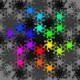# automatic zordering with plotsThe zorder-option can be used to specify on which layer the elements of a plot are drawn. Is there a way to automatically increase the z-order at each time an element is added to the current Graphics-object? (manually inserting zorder=... is not the solution I want, since it is inflexible (changing the code; shifting code-blocks around))

E.g.

g = Graphics()
g += point((0,0))  # I want zorder=1
g += point((1,0))  # I want zorder=2
# ...

edit retag close merge delete

Sort by » oldest newest most voted

If I understand what you want to do, you would have to have some keyword in order to do this anyway. I suppose you would want something like

sage: G = Graphics()
sage: L = [list of graphics objects you want to add to G]
sage: for g in L:
....:     current_max_zorder = G.get_max_zorder()
....:     G += g.set_zorder(current_max_zorder+1)


Or something similar if you have one graphics object with many primitives that you want to add one at a time. The problem is of course getting current_max_zorder. Here is one way you might do that.

sage: G = plot(sin)+circle((3,2),2)+arrow((5,6),(3,2))
sage: current_max_zorder=0
sage: for g in G:
....:     current_max_zorder=max(current_max_zorder,g.options().get('zorder',current_max_zorder))
sage: current_max_zorder
5


You need this because most graphics objects do not acquire a zorder immediately, though some do in their options decorator. (That is something that could eventually be unified...)

If you think that this is useful enough for everyone, we could open a ticket for making it easier.

morekcrisman's second approach, basically fixing the zorder after the fact, makes the most sense to me. Simply add as you like and then set the zorders from the order in the G list before printing. (I don't think G does any reshuffling, although I could be wrong about that.)

Whatever you do, don't do this.

# monkey madness
zorders = list(g.options().get('zorder') for g in args)
max_zorder = max(zorders) if zorders else 0
for i, p in enumerate(args,1):
p.set_zorder(max_zorder+i)
return f(*args)

# it's asking -- no, begging! -- for all kinds of disaster, and for zero benefit

# seriously, I mean it
G = Graphics()
G += plot(sin) + circle((3,2),2)+ arrow((5,6),(3,2))
G += point((3,4))
print list(g.options()['zorder'] for g in G)

# You are likely to be eaten by a grue.

more

Umm, I'm not sure whether this was sarcastic. I agree that one doesn't want to mess with double-underscore methods lightly, but maybe I'm missing some pop-culture reference here? We do use decorators all the time, which is basically what you are doing here, right?

There's no sarcasm at all on my end: monkeypatching is fun but when I don't understand the underlying code -- and here I don't -- it's simply a good way to have weird and hard-to-diagnose bugs show up. For example, I have no idea if some plotting routine depends on the zorders not being changed while it's working. Probably not, but those are dangerous assumptions to make, and I've burned myself in the past.. Fixing the zorder externally is both simpler and safer.